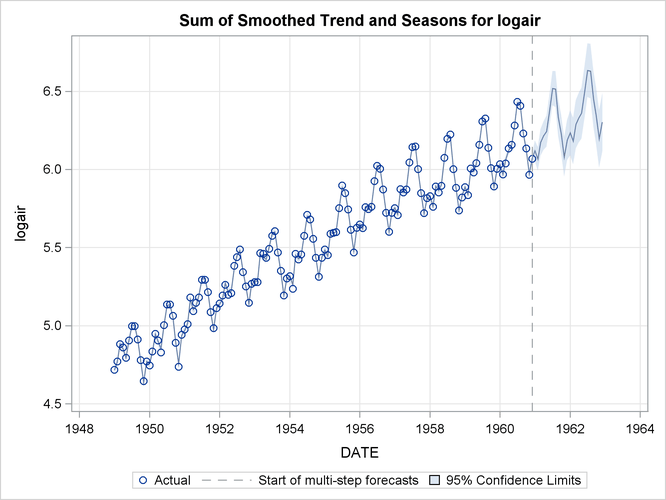The UCM Procedure

A Seasonal Series with Linear Trend

The airline passenger series, given as Series G in Box and Jenkins (1976), is often used in time series literature as an example of a nonstationary seasonal time series. This series is a monthly series consisting of the number of airline passengers who traveled during the years 1949 to 1960. Its main features are a steady rise in the number of passengers from year to year and the seasonal variation in the numbers during any given year. It also exhibits an increase in variability around the trend. A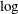transformation is used to stabilize this variability. The following DATA step prepares the-transformed passenger series analyzed in this example:

data seriesG;
set sashelp.air;
logair = log( air );
run;


The following statements produce a time series plot of the series by using the TIMESERIES procedure (see Chapter 32: The TIMESERIES Procedure). The trend and seasonal features of the series are apparent in the plot in Figure 34.1.

proc timeseries data=seriesG plot=series;
id date interval=month;
var logair;
run;


Figure 34.1: Series Plot of Log-Transformed Airline Passenger Series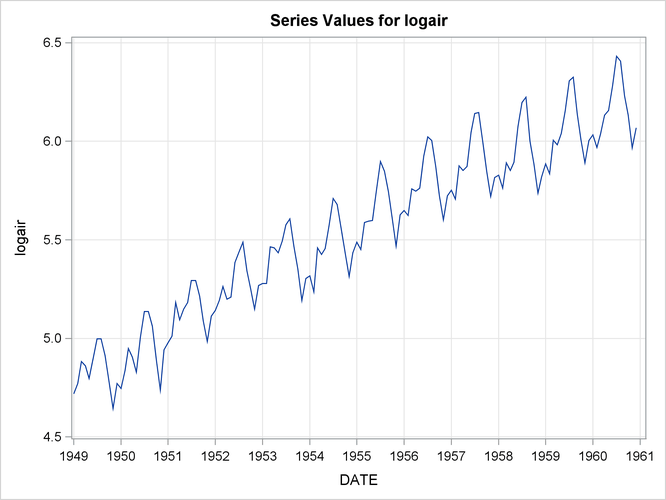In this example this series is modeled using an unobserved component model called the basic structural model (BSM). The BSM models a time series as a sum of three stochastic components: a trend component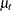, a seasonal component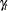, and random error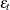. Formally, a BSM for a response series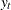can be described as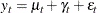Each of the stochastic components in the model is modeled separately. The random error, also called the irregular component, is modeled simply as a sequence of independent, identically distributed (i.i.d.) zero-mean Gaussian random variables. The trend and the seasonal components can be modeled in a few different ways. The model for trend used here is called a locally linear time trend. This trend model can be written as follows: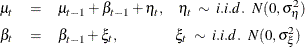These equations specify a trend where the levelas well as the slope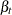is allowed to vary over time. This variation in slope and level is governed by the variances of the disturbance terms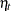and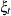in their respective equations. Some interesting special cases of this model arise when you manipulate these disturbance variances. For example, if the variance ofis zero, the slope will be constant (equal to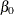); if the variance ofis also zero,will be a deterministic trend given by the line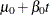. The seasonal model used in this example is called a trigonometric seasonal. The stochastic equations governing a trigonometric seasonal are explained later (see the section Modeling Seasons). However, it is interesting to note here that this seasonal model reduces to the familiar regression with deterministic seasonal dummies if the variance of the disturbance terms in its equations is equal to zero. The following statements specify a BSM with these three components:

proc ucm data=seriesG;
id date interval=month;
model logair;
irregular;
level;
slope;
season length=12 type=trig print=smooth;
estimate;
run;


The PROC UCM statement signifies the start of the UCM procedure, and the input data set, seriesG, containing the dependent series is specified there. The optional ID statement is used to specify a date, datetime, or time identification variable, date in this example, to label the observations. The INTERVAL=MONTH option in the ID statement indicates that the measurements were collected on a monthly basis. The model specification begins with the MODEL statement, where the response series is specified (logair in this case). After this the components in the model are specified using separate statements that enable you to control their individual properties. The irregular componentis specified using the IRREGULAR statement and the trend componentis specified using the LEVEL and SLOPE statements. The seasonal componentis specified using the SEASON statement. The specifics of the seasonal characteristics such as the season length, its stochastic evolution properties, etc., are specified using the options in the SEASON statement. The seasonal component used in this example has a season length of 12, corresponding to the monthly seasonality, and is of the trigonometric type. Different types of seasonals are explained later (see the section Modeling Seasons).

The parameters of this model are the variances of the disturbance terms in the evolution equations of,, andand the variance of the irregular component. These parameters are estimated by maximizing the likelihood of the data. The ESTIMATE statement options can be used to specify the span of data used in parameter estimation and to display and save the results of the estimation step and the model diagnostics. You can use the estimated model to obtain the forecasts of the series as well as the components. The options in the individual component statements can be used to display the component forecasts—for example, PRINT=SMOOTH option in the SEASON statement requests the displaying of smoothed forecasts of the seasonal component. The series forecasts and forecasts of the sum of components can be requested using the FORECAST statement. The option PRINT=DECOMP in the FORECAST statement requests the printing of the smoothed trendand the trend plus seasonal component (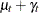).

The parameter estimates for this model are displayed in Figure 34.2.

Figure 34.2: BSM for the Logair Series

The UCM Procedure

Final Estimates of the Free Parameters
Component Parameter Estimate Approx
Std Error
t Value Approx
Pr > |t|
Irregular Error Variance 0.00023436 0.0001079 2.17 0.0298
Level Error Variance 0.00029828 0.0001057 2.82 0.0048
Slope Error Variance 8.47916E-13 6.2271E-10 0.00 0.9989
Season Error Variance 0.00000356 1.32347E-6 2.69 0.0072

The estimates suggest that except for the slope component, the disturbance variances of all the components are significant—that is, all these components are stochastic. The slope component, however, appears to be deterministic because its error variance is quite insignificant. It might then be useful to check if the slope component can be dropped from the model—that is, if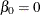. This can be checked by examining the significance analysis table of the components given in Figure 34.3.

Figure 34.3: Component Significance Analysis for the Logair Series

Significance Analysis of Components (Based
on the Final State)
Component DF Chi-Square Pr > ChiSq
Irregular 1 0.08 0.7747
Level 1 117867 <.0001
Slope 1 43.78 <.0001
Season 11 507.75 <.0001

This table provides the significance of the components in the model at the end of the estimation span. If a component is deterministic, this analysis is equivalent to checking whether the corresponding regression effect is significant. However, if a component is stochastic, then this analysis pertains only to the portion of the series near the end of the estimation span. In this example the slope appears quite significant and should be retained in the model, possibly as a deterministic component. Note that, on the basis of this table, the irregular component’s contribution appears insignificant toward the end of the estimation span; however, since it is a stochastic component, it cannot be dropped from the model on the basis of this analysis alone. The slope component can be made deterministic by holding the value of its error variance fixed at zero. This is done by modifying the SLOPE statement as follows:

  slope variance=0 noest;


After a tentative model is fit, its adequacy can be checked by examining different goodness-of-fit measures and other diagnostic tests and plots that are based on the model residuals. Once the model appears satisfactory, it can be used for forecasting. An interesting feature of the UCM procedure is that, apart from the series forecasts, you can request the forecasts of the individual components in the model. The plots of component forecasts can be useful in understanding their contributions to the series. The following statements illustrate some of these features:

proc ucm data=seriesG;
id date interval = month;
model logair;
irregular;
level plot=smooth;
slope variance=0 noest;
season length=12 type=trig
plot=smooth;
estimate;
run;


The table given in Figure 34.4 shows the goodness-of-fit statistics that are computed by using the one-step-ahead prediction errors (see the section Statistics of Fit). These measures indicate a good agreement between the model and the data. Additional diagnostic measures are also printed by default but are not shown here.

Figure 34.4: Fit Statistics for the Logair Series

The UCM Procedure

Fit Statistics Based on Residuals
Mean Squared Error 0.00147
Root Mean Squared Error 0.03830
Mean Absolute Percentage Error 0.54132
Maximum Percent Error 2.19097
R-Square 0.99061
Random Walk R-Square 0.87288

The first plot, shown in Figure 34.5, is produced by the PLOT=SMOOTH option in the LEVEL statement, it shows the smoothed level of the series.

Figure 34.5: Smoothed Trend in the Logair Series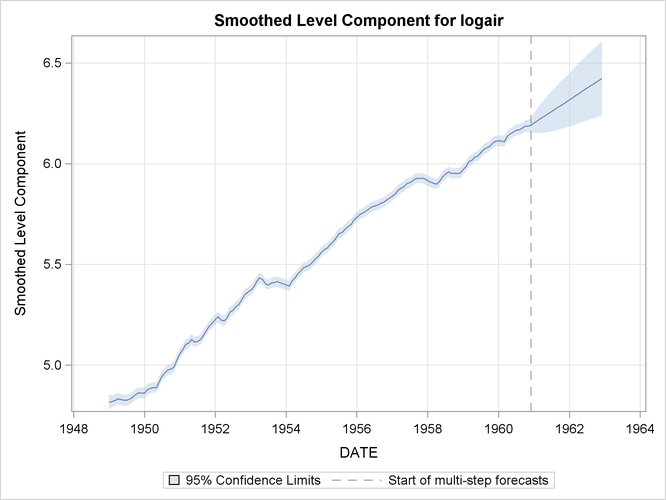The second plot (Figure 34.6), produced by the PLOT=SMOOTH option in the SEASON statement, shows the smoothed seasonal component by itself.

Figure 34.6: Smoothed Seasonal in the Logair Series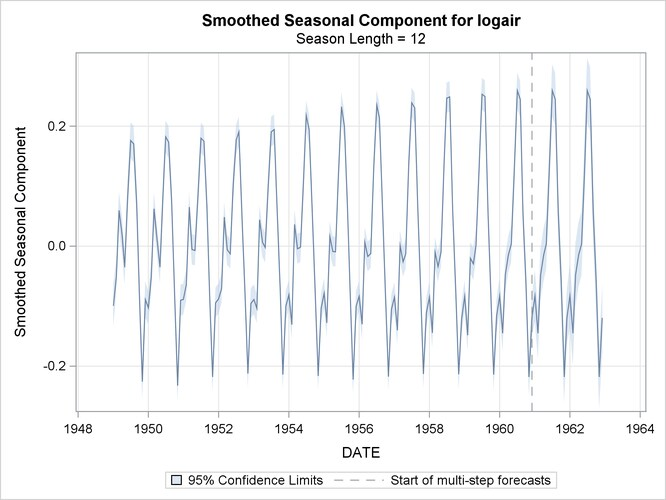The plot of the sum of the trend and seasonal component, produced by the PLOT=DECOMP option in the FORECAST statement, is shown in Figure 34.7. You can see that, at least visually, the model seems to fit the data well. In all these decomposition plots the component estimates are extrapolated for two years in the future based on the LEAD=24 option specified in the FORECAST statement.

Figure 34.7: Smoothed Trend plus Seasonal in the Logair Series